# Degenerate partial differential equation

(diff) ← Older revision | Latest revision (diff) | Newer revision → (diff)

A partial differential equation whose type degenerates in certain points of the domain of definition of the equation or at the boundary of this domain. The type of an equation or of a system of equations at a point is defined by one or more algebraic relations between the coefficients. These relations usually comprise strict inequalities. If, at certain points of the domain under consideration, the inequalities which are satisfied are weak rather than strict, one speaks of degeneration of type, while the equation (or system of equations) is called degenerate. One distinguishes between a degenerate elliptic equation, a degenerate hyperbolic equation and a degenerate parabolic equation (or systems of such equations).

Examples: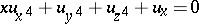is a degenerate elliptic equation in the half-space;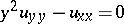is a degenerate hyperbolic equation in the entire plane;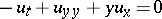is a degenerate parabolic equation in the domain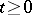;is a degenerate elliptic system for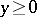.

Degenerate equations are encountered in the theory of boundary layers, in the theory of shells (if the loading does not comprise moments), in the theory of diffusion processes, in particular in the theory of Brownian motion, and in many other problems in physics and mechanics.

Degenerate equations are studied by posing two closely connected problems: 1) a demonstration of the solvability of, say, boundary value problems taking into account changes in their formulation which are a consequence of the degeneration of type; and 2) a determination of properties of the solutions which are analogous to those of non-degenerate equations (smoothness, Harnack inequalities for elliptic and parabolic equations, etc.).

Second-order degenerate equations of elliptic and parabolic types have been most extensively studied; strictly speaking, a parabolic equation may also be considered as a degenerate elliptic equation which satisfies additional conditions. If degeneration of type is not present on the boundary alone but also at interior points (e.g. at all points of the domain in question), such equations might be called equations with a non-negative characteristic form, elliptic-parabolic equations or ultra-parabolic equations.

A characteristic feature of degenerate equations is the specific formulation of boundary value problems. Boundary conditions may be applied not on the boundary as a whole, but on one of its parts only. M.V. Keldysh was the first to note the dependence of the formulation of a boundary value problem on the nature of the degeneration of an elliptic equation on the boundary. For the general second-order elliptic-parabolic equation(*)

the first boundary value problem may be posed as follows. Letbe the boundary of the domainunder consideration, letbe the interior normal to, and let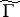be that part ofwhere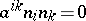and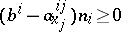. The problem is to find a solution of (*) insuch that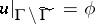. The existence and the uniqueness of the generalized solution of this problem have been proved and sufficiency conditions for the smoothness of the generalized solution have been specified.

Since first-order equations may be regarded as a special case of degenerate equations, it is clear that solutions of degenerate elliptic equations will in general not be smooth within the domain if the boundary conditions are not sufficiently smooth. There are examples showing that even if the boundary conditions and the coefficients of the degenerate equations are infinitely differentiable, their solutions need not be infinitely differentiable. However, the condition for hypo-ellipticity of the general second-order degenerate elliptic equation has been found.

The properties of solutions of second-order degenerate elliptic and parabolic equations can be studied by both geometric and probabilistic methods.

Most of the studies of degenerate hyperbolic equations concern second-order equations with two independent variables which degenerate at the boundary of the domain. These studies were inspired, in the first place, by the study of equations of mixed type and related problems in gas dynamics. To illustrate the problems which arise in this connection, consider the Cauchy problem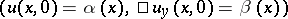for an equation with principal part. If, the problem has a unique solution, and if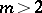the Cauchy problem is usually ill-posed. For an equation with principal part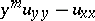the Cauchy problem with data on the line of degeneration is well-posed for. If, the formulation of the problem is usually modified, as in the case of elliptic equations. Instead of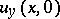one gives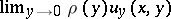, where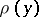is some positive function which depends on the coefficients of the equation.

The well-posedness of Cauchy's problem has been proved, under certain conditions, for hyperbolic equations in more spatial variables,which become degenerate both on the initial plane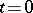and inside the domain. The most important condition here is the validity of the inequalitywhereandare certain positive constants.

A number of results obtained for quasi-linear degenerate equations are also applicable to non-linear equations.

How to Cite This Entry:
Degenerate partial differential equation. Encyclopedia of Mathematics. URL: http://encyclopediaofmath.org/index.php?title=Degenerate_partial_differential_equation&oldid=46615
This article was adapted from an original article by A.M. Il'in (originator), which appeared in Encyclopedia of Mathematics - ISBN 1402006098. See original article